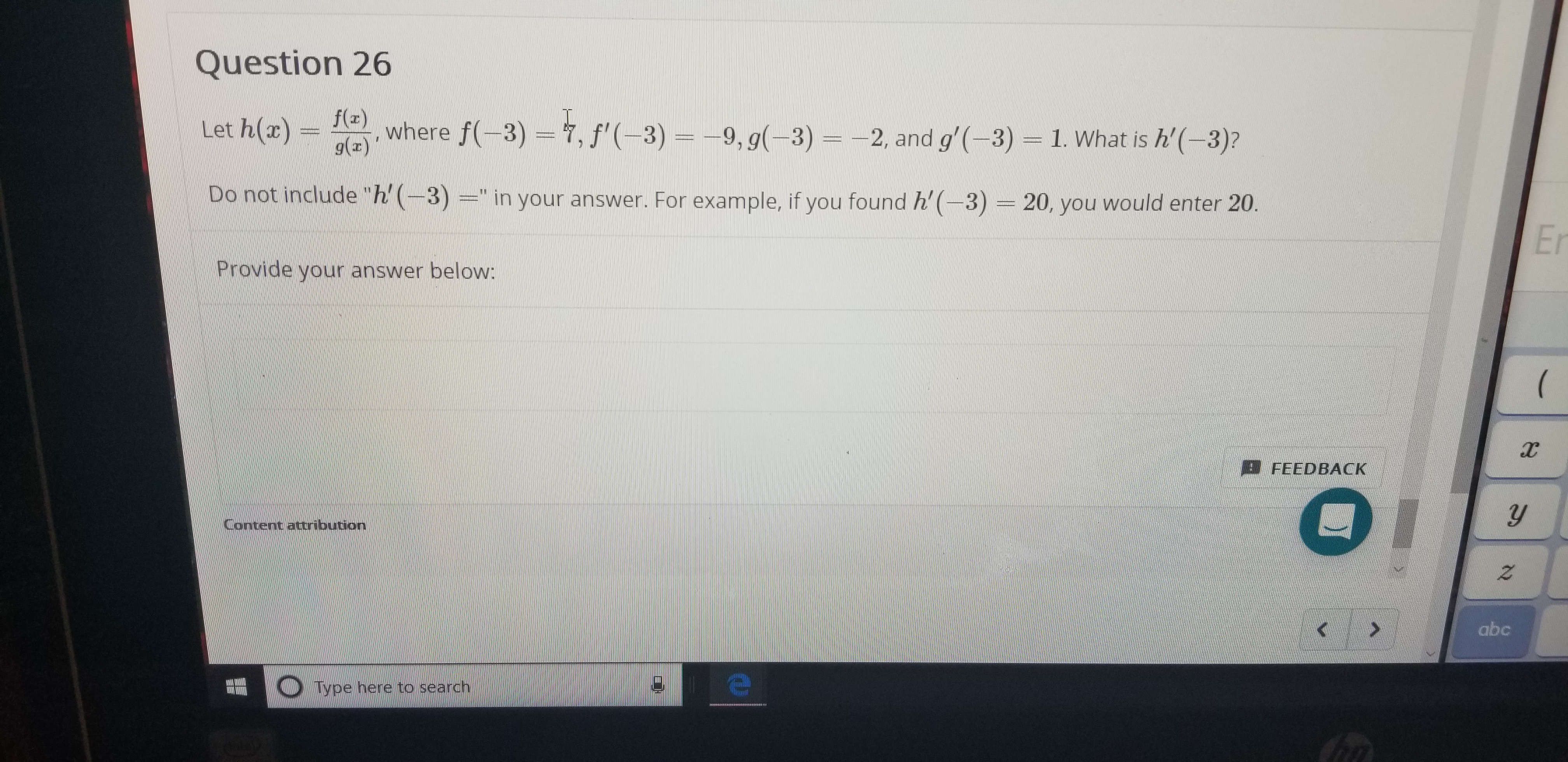# Question 26f(2)where f(-3) 7, f'(-3)- 9, g(-3) =-2, and g'(-3)g()Let h(ax)1. What is h'(-3)?Do not include "h(3)=" in your answer. For example, if you found h'(-3)= 20, you would enter 20.ErProvide your answer below(FEEDBACKyContent attribution2abcO Type here to searchtha

Question
6 viewshelp_outlineImage TranscriptioncloseQuestion 26 f(2) where f(-3) 7, f'(-3)- 9, g(-3) =-2, and g'(-3) g() Let h(ax) 1. What is h'(-3)? Do not include "h(3)=" in your answer. For example, if you found h'(-3)= 20, you would enter 20. Er Provide your answer below ( FEEDBACK y Content attribution 2 abc O Type here to search tha fullscreen
check_circle

Step 1

Let, h(x) = f(x)/g(x).

Find: h’(x).

Step 2

Differentiate h(x) with respect ...

### Want to see the full answer?

See Solution

#### Want to see this answer and more?

Solutions are written by subject experts who are available 24/7. Questions are typically answered within 1 hour.*

See Solution
*Response times may vary by subject and question.
Tagged in

### Derivative